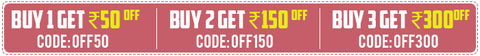# LingerieRs. 199.00
Rs. 499.00
Rs. 199.00
Rs. 499.00
Rs. 599.00
Rs. 999.00
Rs. 599.00
Rs. 999.00
Rs. 599.00
Rs. 999.00
Rs. 699.00
Rs. 1,299.00
Rs. 599.00
Rs. 999.00
Rs. 599.00
Rs. 999.00
Rs. 199.00
Rs. 499.00
Rs. 199.00
Rs. 499.00
Rs. 599.00
Rs. 999.00
Rs. 699.00
Rs. 1,199.00
Rs. 199.00
Rs. 499.00
Rs. 199.00
Rs. 499.00
Rs. 239.00
Rs. 499.00
Rs. 199.00
Rs. 499.00
Rs. 599.00
Rs. 999.00
Rs. 599.00
Rs. 999.00
Rs. 599.00
Rs. 999.00
Rs. 199.00
Rs. 499.00
Rs. 199.00
Rs. 499.00
Rs. 599.00
Rs. 999.00
Rs. 599.00
Rs. 999.00
Rs. 199.00
Rs. 499.00
Rs. 199.00
Rs. 499.00
Rs. 199.00
Rs. 499.00
Rs. 199.00
Rs. 499.00
Rs. 239.00
Rs. 499.00
Rs. 239.00
Rs. 499.00
Rs. 599.00
Rs. 999.00
Rs. 599.00
Rs. 999.00
Rs. 599.00
Rs. 999.00
Rs. 499.00
Rs. 999.00